# 6.11: Exercises

•• Anonymous
• LibreTexts
$$\newcommand{\vecs}{\overset { \rightharpoonup} {\mathbf{#1}} }$$ $$\newcommand{\vecd}{\overset{-\!-\!\rightharpoonup}{\vphantom{a}\smash {#1}}}$$$$\newcommand{\id}{\mathrm{id}}$$ $$\newcommand{\Span}{\mathrm{span}}$$ $$\newcommand{\kernel}{\mathrm{null}\,}$$ $$\newcommand{\range}{\mathrm{range}\,}$$ $$\newcommand{\RealPart}{\mathrm{Re}}$$ $$\newcommand{\ImaginaryPart}{\mathrm{Im}}$$ $$\newcommand{\Argument}{\mathrm{Arg}}$$ $$\newcommand{\norm}{\| #1 \|}$$ $$\newcommand{\inner}{\langle #1, #2 \rangle}$$ $$\newcommand{\Span}{\mathrm{span}}$$ $$\newcommand{\id}{\mathrm{id}}$$ $$\newcommand{\Span}{\mathrm{span}}$$ $$\newcommand{\kernel}{\mathrm{null}\,}$$ $$\newcommand{\range}{\mathrm{range}\,}$$ $$\newcommand{\RealPart}{\mathrm{Re}}$$ $$\newcommand{\ImaginaryPart}{\mathrm{Im}}$$ $$\newcommand{\Argument}{\mathrm{Arg}}$$ $$\newcommand{\norm}{\| #1 \|}$$ $$\newcommand{\inner}{\langle #1, #2 \rangle}$$ $$\newcommand{\Span}{\mathrm{span}}$$$$\newcommand{\AA}{\unicode[.8,0]{x212B}}$$

#### 6.2: Molecular Mass and Formula Mass

1. What is the total mass (amu) of carbon in each of the following molecules?
1. CH4
2. CHCl3
3. C12H10O6
4. CH3CH2CH2CH2CH3
1. 12.01 amu
2. 12.01 amu
3. 144.1 amu
4. 60.05 amu

1. What is the total mass of hydrogen in each of the molecules?
1. CH4
2. CHCl3
3. C12H10O6
4. CH3CH2CH2CH2CH3
1. 4.032 amu
2. 1.008 amu
3. 10.08 amu
4. 12.10 amu

1. Calculate the molecular or formula mass of each of the following:
1. P4
2. H2O
3. Ca(NO3)2
4. CH3CO2H (acetic acid)
5. C12H22O11 (sucrose, cane sugar)
1. 123.9 amu
2. 18.02 amu
3. 164.10 amu
4. 60.05 amu
5. 342.3 amu

1. Determine the molecular mass of the following compounds:
1.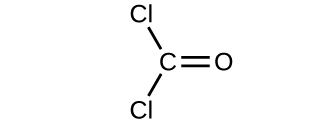2.3.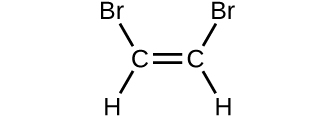4.1. 98.91 amu
2. 26.04 amu
3. 185.8 amu
4. 98.09 amu

1. Determine the molecular mass of the following compounds:
1.2.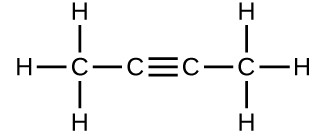3.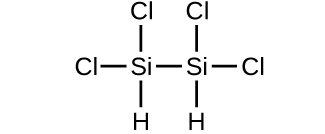4.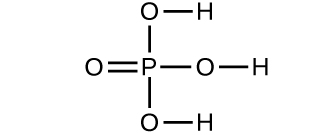1. 56.10 amu
2. 54.09 amu
3. 200.0 amu
4. 97.99 amu

1. Which molecule has a molecular mass of 28.05 amu?
1.2.3.molecule b

#### 6.3: Counting Objects By Weighing

1. Compare 1 mole of H2, 1 mole of O2, and 1 mole of F2.
1. Which has the largest number of molecules? Explain why.
2. Which has the greatest mass? Explain why.
1. They all have the same number of molecules. One mole is 6.022 × 1023 particles, regardless of the identity of the particle.
2. The F2 will have the greatest mass because a molecule of F2 weighs more than a molecule of O2 or H2.

#### 6.4: Molar Mass

1. How are the molecular mass and the molar mass of a compound similar and how are they different?

The two masses have the same numerical value, but the units are different: the molecular mass is the mass of 1 molecule in amu while the molar mass is the mass of 6.022 × 1023 molecules in grams.

1. Determine the molar mass of each substance.
1. Si
2. SiH4
3. K2O
1. 28.09 g
2. 32.12 g
3. 94.20 g

1. Determine the molar mass of each substance.
1. Cl2
2. SeCl2
3. Ca(C2H3O2)2
1. 70.90 g
2. 149.86 g
3. 158.17 g

1. Determine the molar mass of each substance.
1. Al
2. Al2O3
3. CoCl3
1. 26.98 g
2. 101.96 g
3. 165.3 g

1. Calculate the molar mass of each of the following compounds:
1. hydrofluoric acid, HF
2. ammonia, NH3
3. nitric acid, HNO3
4. silver sulfate, Ag2SO4
5. boric acid, B(OH)3
1. 20.01 g
2. 17.03 g
3. 63.02 g
4. 311.81 g
5. 61.83 g

1. Calculate the molar mass of each of the following minerals:
1. limestone, CaCO3
2. halite, NaCl
3. beryl, Be3Al2Si6O18
4. malachite, Cu2(OH)2CO3
5. turquoise, CuAl6(PO4)4(OH)8(H2O)4
1. 100.09 g
2. 58.44 g
3. 537.5 g
4. 813.4 g

1. Calculate the molar mass of each of the following:
1. the anesthetic halothane, C2HBrClF3
2. the herbicide paraquat, C12H14N2Cl2
3. caffeine, C8H10N4O2
4. urea, CO(NH2)2
5. a typical soap, C17H35CO2Na
1. 197.38 g
2. 257.2 g
3. 194.20 g
4. 60.06 g
5. 306.5 g

#### 6.5: Mole Calculations

1. What is the mass of 4.44 mol of Mn?

244 g

1. What is the mass of 0.311 mol of Xe?

40.8 g

1. What is the mass of 12.34 mol of Al2(SO4)3?

4,222 g

1. What is the mass of 0.0656 mol of PbCl2?

18.2 g

1. How many moles are present in 45.6 g of CO?

1.63 mol

1. How many moles are present in 0.00339 g of LiF?

1.31 × 10−4 mol

1. How many moles are present in 1.223 g of SF6?

8.37 × 10−3 mol

1. How many moles are present in 48.8 g of BaCO3?

0.247 mol

1. Determine the mass of each of the following:
1. 2.345 mol LiCl
2. 0.0872 mol acetylene, C2H2
3. 3.3 × 10−2 mol Na2CO3
4. 1.23 × 103 mol fructose, C6H12O6
5. 0.5758 mol FeSO4(H2O)7
1. 99.40 g
2. 2.27 g
3. 3.5 g
4. 2.22 × 105 g
5. 160.1 g

1. The approximate minimum daily dietary requirement of the amino acid leucine, C6H13NO2, is 1.1 g. What is this requirement in moles?

8.4 × 10−3 mol

1. Determine the mass in grams of each of the following:
1. 0.600 mol of oxygen atoms
2. 0.600 mol of oxygen molecules, O2
3. 0.600 mol of ozone molecules, O3
1. 9.60 g
2. 19.2 g
3. 28.8 g

1. How many moles are present in 54.8 mL of mercury if the density of mercury is 13.6 g/mL?

3.72 mol

1. How many moles are present in 56.83 mL of O2 if the density of O2 is 0.00133 g/mL?

2.36 × 10−3 mol

#### 6.6: Counting Particles By Weighing

1. How many atoms are present in 4.55 mol of Fe?

2.740 × 1024 atoms

1. How many atoms are present in 0.0665 mol of K?

4.00 × 1022 atoms

1. How many molecules are present in 2.509 mol of H2S?

1.511 × 1024 molecules

1. How many molecules are present in 0.336 mol of acetylene (C2H2)?

2.02 × 1023 molecules

1. How many moles are present in 3.55 × 1024 Pb atoms?

5.90 mol

1. How many moles are present in 2.09 × 1022 Ti atoms?

0.0347 mol

1. How many moles are present in 1.00 × 1023 PF3 molecules?

0.166 mol

1. How many moles are present in 5.52 × 1025 penicillin molecules?

91.7 mol

1. How many moles are present in 6.411 kg of CO2? How many molecules is this?

145.7 mol; 8.772 × 1025 molecules

1. How many moles are present in 2.998 mg of SCl4? How many molecules is this?

1.724 × 10−5 mol; 1.038 × 1019 molecules

1. What is the mass in milligrams of 7.22 × 1020 molecules of CO2?

52.8 mg

1. What is the mass in kilograms of 3.408 × 1025 molecules of SiS2?

5.220 kg

1. What is the mass in grams of 1 molecule of H2O?

2.992 × 10−23 g

1. What is the mass in grams of 1 atom of Al?

4.480 × 10−23 g

1. What is the volume in mL of 3.44 mol of Ga if the density of Ga is 6.08 g/mL?

39.4 mL

1. What is the volume in L of 0.662 mol of He if the density of He is 0.1785 g/L?

14.8 L

1. A 55-kg woman has 7.5 × 10−3 mol of hemoglobin (molar mass = 64,456 g/mol) in her blood. How many hemoglobin molecules is this? What is this quantity in grams?

4.5 × 1021 molecules; 4.8 × 102 g

1. Diamond is one form of elemental carbon. An engagement ring contains a diamond weighing 1.25 carats (1 carat = 200 mg). How many atoms are present in the diamond?

1.25 × 1022 atoms

1. The Cullinan diamond was the largest natural diamond ever found (January 25, 1905). It weighed 3104 carats (1 carat = 200 mg). How many carbon atoms were present in the stone?

3.113 × 1025 atoms

1. One 55-gram serving of a particular cereal supplies 270 mg of sodium, 11% of the recommended daily allowance. How many moles and atoms of sodium are in the recommended daily allowance?

0.012 mol; 7.1 × 1021 atoms

1. A certain nut crunch cereal contains 11.0 grams of sugar (sucrose, C12H22O11) per serving size of 60.0 grams. How many servings of this cereal must be eaten to consume 0.0278 moles of sugar?

0.865 servings

1. Which of the following represents the least number of molecules?
1. 20.0 g of H2O (18.02 g/mol)
2. 77.0 g of CH4 (16.06 g/mol)
3. 68.0 g of CaH2 (42.09 g/mol)
4. 100.0 g of N2O (44.02 g/mol)
5. 84.0 g of HF (20.01 g/mol)

20.0 g H2O

#### 6.7: Using Chemical Formulas as Conversion Factors

1. Which contains the greatest mass of oxygen: 0.75 mol of ethanol (C2H5OH), 0.60 mol of formic acid (HCO2H), or 1.0 mol of water (H2O)? Explain why.

Formic acid. Its formula has twice as many oxygen atoms as the other two compounds (one each). Therefore, 0.60 mol of formic acid would be equivalent to 1.20 mol of a compound containing a single oxygen atom.

1. Which contains the greatest number of moles of oxygen atoms: 1 mol of methanol (CH3OH), 1 mol of acetic acid (CH3CO2H), or 1 mol of glycerol (C3H8O3)? Explain why.

Glycerol. Each molecule of glycerol has three atoms of oxygen as compared to one atom of oxygen in a molecule of methanol and two atoms of oxygen in acetic acid.

1. Determine the number of moles of the compound and determine the number of moles of each type of atom in each of the following:
1. 2.12 g of potassium bromide, KBr
2. 0.1488 g of phosphoric acid, H3PO4
3. 23 kg of calcium carbonate, CaCO3
4. 78.452 g of aluminum sulfate, Al2(SO4)3
5. 0.1250 mg of caffeine, C8H10N4O2
1. 0.0178 mol KBr; 0.0178 mol K; 0.0178 mol Br
2. 1.478 × 10−3 mol H3PO4; 4.433 × 10−3 mol H; 1.478 × 10−3 mol P; 5.911 × 10−3 mol O
3. 2.3 × 102 mol CaCO3; 2.3 × 102 mol Ca; 2.3 × 102 mol C; 6.9 × 102 mol O
4. 0.2293 mol Al2(SO4)3; 0.4586 mol Al; 0.6878 mol S; 2.751 mol O
5. 6.437 × 10−7 mol caffeine; 5.149 × 10−6 mol C; 6.437 × 10−6 mol H; 2.575 × 10−6 mol N; 1.287 × 10−6 mol O

1. Determine the number of atoms and the mass of zirconium, silicon, and oxygen found in 0.3384 mol of zircon, ZrSiO4, a semiprecious stone.

zirconium: 2.038 × 1023 atoms; 30.87 g; silicon: 2.038 × 1023 atoms; 9.504 g; oxygen: 8.151 × 1023 atoms; 21.66 g

1. Determine which of the following contains the greatest mass of hydrogen: 1 mol of CH4, 0.6 mol of C6H6, or 0.4 mol of C3H8.

1 mol CH4

1. Determine which of the following contains the greatest mass of aluminum: 122 g of AlPO4, 266 g of Al2Cl6, or 225 g of Al2S3.

225 g Al2S3

1. A tube of toothpaste contains 0.76 g of sodium monofluorophosphate (Na2PO3F) in 100 mL.
1. What mass of fluorine atoms in mg was present?
2. How many fluorine atoms were present?
1. 1.0 × 102 mg F
2. 3.2 × 1021 atoms F

#### 6.8: Percent-By-Mass Composition

1. A 125 g sample of a compound known to contain only phosphorus and oxygen was shown to contain 54.5 g of phosphorus. What is the percent by mass composition of the compound?

43.6% P; 56.4% O

1. A compound containing only carbon and hydrogen was submitted for analysis. A 1.237 g sample of the compound was found to contain 1.140 g of carbon. What is the percent composition of the compound?

92.16% C; 7.84% H

1. Dichloroethane, a compound that is often used for dry cleaning, contains carbon, hydrogen, and chlorine. A 14.83 g sample of dichloroethane contained 3.60 g of carbon and 0.61 g of hydrogen. What is the percent by mass composition of dichloroethane?

24.3% C; 4.1% H; 71.6% Cl

1. A major textile dye manufacturer developed a new yellow dye containing carbon, nitrogen, and hydrogen. When 16.94 g of the dye was analyzed, it was found to contain 3.00 g of nitrogen and 1.07 g of hydrogen. What is the percent composition of the dye?

75.98% C; 17.7% N; 6.32% H

#### 6.9: Mass Percent Composition from a Chemical Formula

1. Calculate the following to four significant figures:
1. the percent composition of ammonia, NH3
2. the percent composition of photographic “hypo,” Na2S2O3
3. the percent of calcium ion in Ca3(PO4)2
1. 82.25% N; 17.75% H
2. 29.08% Na; 40.56% S; 30.36% O
3. 38.76% Ca2+

1. Determine the following to four significant figures:
1. the percent composition of hydrazoic acid, HN3
2. the percent composition of TNT, C6H2(CH3)(NO2)3
3. the percent of SO42 in Al2(SO4)3
1. 2.342% H; 97.66% N
2. 37.01% C; 2.219% H; 18.50% N; 42.26% O
3. 84.23% SO42

1. Determine the percent ammonia, NH3, in Co(NH3)6Cl3, to three significant figures.

38.2% NH3

1. Determine the percent water in CuSO4∙5H2O to three significant figures.

36.1% H2O

1. Copper(I) iodide (CuI) is often added to table salt as a dietary source of iodine. How many moles of CuI are contained in 1.00 lb (454 g) of table salt containing 0.0100% CuI by mass?

2.38 × 10−4 mol CuI

1. A cough syrup contains 5.0% ethyl alcohol, C2H5OH, by mass. If the density of the solution is 0.9928 g/mL, how many grams of ethyl alcohol are contained in a 20.0 mL dose?

0.99 g ethyl alcohol

1. D5W is a solution used as an intravenous fluid. It is a 5.0% by mass solution of dextrose, C6H12O6, in water. If the density of D5W is 1.029 g/mL, calculate the mass of dextrose in a 1.00 L IV fluid bag.

51 g dextrose

1. Find the mass of sulfuric acid in 325 mL of a 40.0% by mass aqueous solution of sulfuric acid, H2SO4, for which the density is 1.3057 g/mL.

1.70 × 102 g H2SO4

This page was adapted from "Beginning Chemistry (Ball)" by LibreTexts and "Chemistry (OpenStax)" by LibreTexts and is shared under a CC BY-NC-SA 4.0 license and was authored, remixed, and/or curated by Vicki MacMurdo (Anoka-Ramsey Community College) and Lance S. Lund (Anoka-Ramsey Community College).

This page titled 6.11: Exercises is shared under a CC BY-NC-SA 4.0 license and was authored, remixed, and/or curated by Anonymous.